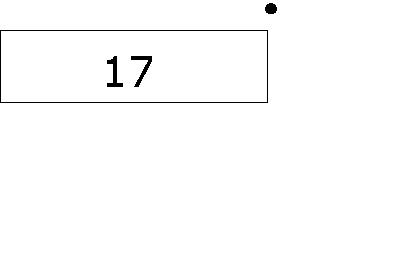# Basic Comparison Model

Here are the 2 basic models. You can also check out the other variations of the comparison model by clicking the links below.

(i) Variations of "More than" Model

_________ more than 17 is 21.

4 more than _________ is 21.

_________ is 4 more than 17.

21 is 4 more than __________.

21 is __________ more than 17.

(i) Variations of "Less than" Model

__________ less than 17 is 13.

4 less than __________ is 13.

__________ is 4 less than 17.

13 is 4 less than __________.

13 is __________ less than 17.

There are always three numerical values in such "more than" and "less than" questions, i.e., "4", "17" and "?".

(a) "More than" Model

Step 1: Draw a box and write the number found after the word "than" in this box.Step 2: Draw a dot at the top-right corner of the first box. This marks the starting point of the arrow we are about to draw next.Step 3: Draw an arrow from the dot pointing to the right. (The arrow points to the right because it is a "more than" question.Step 4: Since it is "more than", we are actually making the number 17 (starting number) greater by 4. Hence, draw a second box directly below the arrow to show that the number 17 has been lengthened by 4.Step 5: Draw a last box to match the length of the first two boxes and write the unknown number (?) in this box.From the model,

17 + 4 = ?
17 + 4 = 21

(b) "Less than" Model

Step 1: Draw a box and write the number found after the word "than" in this box.Step 2: Draw a dot at the top-right corner of the first box. This marks the starting point of the arrow we are about to draw next.Step 3: Draw an arrow from the dot pointing to the left. (The arrow points to the left because it is a "less than" question.Step 4: Since it is "less than", we are actually making the number 17 (starting number) smaller by 4. Hence, draw a second box directly below the arrow and the first box to show that the number 17 has been shortened by 4.Step 5: Draw a last box next to the second box to make the model form a rectangle and write the unknown number (?) in this box.From the model,

17 - 4 = ?
17 - 4 = 13